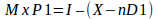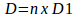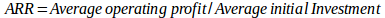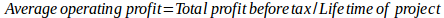# How to Calculate Pay Back Period

## Dividend Decision

The dividend is the amount of money paid by the company to its investors. The dividend payments are calculated as dividend per share multiply by a number of outstanding shares.Assignment:

The machine cost Rs. 1,00,000 and has a scrap value of Rs. 10,000 after 5 years. The
net profits before depreciation and taxes for the five years period are to be projected
that Rs. 20,000, Rs. 24,000, Rs. 30,000, Rs. 26,000 and Rs. 22,000. Taxes are
50%. Calculate pay-back period and accounting rate of return.

Using the MM approach, the price of the share (P1) at the end of the period will be defined as a following:Where,

P0 = Prevailing market price of a share

K e = Cost of equity capital

D1 = Dividend at the end of the first period

1. Calculation price of a share at the end of the period when dividend is not declared

If the dividends are not declared, the company hasn’t any obligation to its investors, and the dividends will be equal zero.

P1 = Rs.100 x (1+0.15)-0 = Rs.115

Price of a share at the end of the period when dividend declaration is avoided is Rs. 115.

1. Calculation price of a share at the end of the period when dividend is declared

If the dividends are declared of Rs. 15, the price of a share at the end of the period will be calculated as a following:

P1 = Rs.100 x (1+0.15)-15 = Rs.100

Price of a share at the end of the period when dividend declaration is present is Rs. 100.

1. Finding out the number of new share for new investment of Rs.15,00,000

For finding the required number of share for investment need, the formula will be used:Where,

M = Number of new shares to be issued

P1 = Price at which new issue is to be made

I = Amount of investment required

X = Net profit earned for period

nD1 = Dividend paid for the period

Step 1

Using the data of requirement #b, the price of share at which new shares will be issued equals R s.100

Step 2

The annual dividend (D) will be calculated using the following formula:Where

n = Number of outstanding shares at April 1

D1 = Dividend per share

D =25,000 x Rs.15 =Rs.3,75,000

Step 3

If the required investment equals Rs.15,00,000 and net income for the period is Rs.7,50,000, the number of new share for new investment (M) will be computed:M = (15,00,000-(7,50,000-3,75,000))/100 = 11,25,000/100 = 11,250

The number of new share for the new investment of Rs.15,00,000 equal 11,250 shares.

## Capital Budgeting

Calculation payback period

The payback period is the period of time when the future cash inflows will cover the initial outlay of the project.

Step 1: Calculation of the annual depreciation

Look at these data:

 Initial cash outflow (Rs.) 100,000 Less: Scrap value (Rs.) 10,000 Net initial cash outflow (Rs.) 90,000 Divided by: Estimated life of the depreciation, years 5 Depreciation (Rs.) 18,000

Step 2: Calculation of Cash Inflow

The cash inflow is calculated as the summary of net income and depreciation.

The net income is defined as excluding from the profit before depreciation and tax less depreciation and excluding the amount of tax.

 Year 0 1 2 3 4 5 Profit before depreciation and tax 20,000 24,000 30,000 26,000 22,000 Less: Depreciation 18,000 18,000 18,000 18,000 18,000 Profit before tax 2,000 6,000 12,000 8,000 4,000 Less: Tax -50% 50% 1,000 3,000 6,000 4,000 2,000 Net Income 1,000 3,000 6,000 4,000 2,000 Add: Depreciation 18,000 18,000 18,000 18,000 18,000 Cash Inflow 19,000 21,000 24,000 22,000 20,000

Step 3: Calculation of payback period

The calculation of payback period if the annual cash inflows are not equal amounts is presented in the table below using the cumulative cash inflows.

 Year 1 2 3 4 5 Cash Inflow 19,000 21,000 24,000 22,000 20,000 Cumulative Cash Inflow 19,000 40,000 64,000 86,000 106,000

If the net initial cost of equipment of Rs.90,000, the pay-back period will be 4 years and the remaining of Rs.4,000 in 3months (4,000/20,000) when the cumulative cash inflows will cover the initial investment.

Pay-back period is 4 years and 3 months.

## Calculation Accounting Rate of Return

The accounting rate of return (ARR) is the average operating profit or profit before tax divided by the average initial investment.Where,Average net income = (2,000+6,000+12,000+8,000+4,000)/5 =32,000/5 =6,400

Average investment = (1,00,000 +10,000)/2 =55,000

Accounting rate of return =6,400/55,000 =0.1162 or 11.62%

Accounting rate of return equals 11.62%.

Having proven our theoretical and practical competence, we are glad to introduce you to our services.